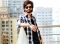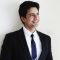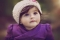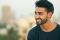## How can I reimplement JavaScript delete method?Updated on 08-Dec-2022 09:38:12
The delete operator in JavaScript is used to remove properties from an object to remove element(s) from an array or to remove element(s) from a set object. Always remember the following points about delete: delete is a keyword in JavaScript, so it shouldn’t be altered, and you cannot use delete objects in single variables since it is used only for deleting properties of objects. Let's look in detail at how we can implement delete operators in JavaScript. Deleting object properties Objects in JavaScript, we will write using key-value pair like const Employee = { firstname: 'Devika', ... Read More

## What are Logical Operators in JavaScript?Updated on 13-Jun-2020 12:55:29
JavaScript supports the following logical operators. Assume variable A holds 10 and variable B holds 20, then, Sr.NoOperator and Description1&& (Logical AND)If both the operands are non-zero, then the condition becomes true.Ex: (A && B) is true.2|| (Logical OR)If any of the two operands are non-zero, then the condition becomes true.Ex: (A || B) is true.3! (Logical NOT)Reverses the logical state of its operand. If a condition is true, then the Logical NOT operator will make it false.Ex: ! (A && B) is false.ExampleYou can try the following code to learn how to implement Logical Operators in JavaScript −Live Demo   ... Read More

## How do I create dynamic variable names inside a JavaScript loop?Updated on 20-Jul-2022 08:30:09
In this tutorial, we will use the dynamic variable names inside the JavaScript loop. The dynamic variables name means it is not hardcoded already, and we can create it according to our needs from some other string values.In JavaScript, there are not many applications of the dynamic variable, but it is needed while developing the real-time application. For example, while developing any application, the programmer wants to store any user-related entity to the variable with the same name as the username. In this scenario, programmers need to create dynamic variables inside JavaScript.Users can also refer to the below examples to ... Read More

## Which data is stored in var and how its content is changed in JavaScript?Updated on 13-Sep-2019 07:26:07
JavaScript variables are not typed but their values do have a type. The same variable can be assigned new values.ExampleLive Demo                    var a;          document.write(typeof a+"\r");          a = true;          document.write(typeof a+"\r");          a = 5;          document.write(typeof a+"\r");          a = "web";          document.write(typeof a+"\r");

## What are Comparison Operators in JavaScript?Updated on 13-Sep-2019 07:28:50
JavaScript supports the following comparison operators. Assume variable A holds 10 and variable B holds 20, then:Sr. NoOperator and Description1= = (Equal)Checks if the value of two operands are equal or not, if yes, then the condition becomes true.Ex: (A == B) is not true.2!= (Not Equal)Checks if the value of two operands are equal or not, if the values are not equal, then the condition becomes true.Ex: (A != B) is true.3> (Greater than)Checks if the value of the left operand is greater than the value of the right operand, if yes, then the condition becomes true.Ex: (A > ... Read More

## What are Arithmetic Operators in JavaScript?Updated on 13-Sep-2019 07:32:16
JavaScript supports the following arithmetic operators. Assume variable A holds 10 and variable B holds 20, then −Sr.NoOperator and Description1+ (Addition)Adds two operandsEx: A + B will give 302- (Subtraction)Subtracts the second operand from the firstEx: A - B will give -103* (Multiplication)Multiply both operandsEx: A * B will give 2004/ (Division)Divide the numerator by the denominatorEx: B / A will give 25% (Modulus)Outputs the remainder of an integer divisionEx: B % A will give 06++ (Increment)Increases an integer value by oneEx: A++ will give 117-- (Decrement)Decreases an integer value by oneEx: A-- will give 9The following code shows how ... Read More

## Must you define a data type when declaring a variable in JavaScript?Updated on 03-Jan-2020 10:54:26
In JavaScript, variables are defined using the var keyword followed by the variable name. Before you use a variable in a JavaScript program, you must declare it. Variables are declared with the var keyword as follows.var rank;A data type isn’t needed in JavaScript. Variables in JavaScript are not handled like other strong typed language C++, Java, etc.Storing a value in a variable is called variable initialization. You can do variable initialization at the time of variable creation or at a later point in time when you need that variable.    var name = "Amit";    var rank = 2;

## How are variables allocated memory in JavaScript?Updated on 30-Jul-2019 22:30:21
JavaScript handling of the variable is different from other programming languages C, C++., Java, etc. Variables in JavaScript can be thought of as named containers. You can place data into these containers and then refer to the data simply by naming the container. Before you use a variable in a JavaScript program, you must declare it. Variables are declared with the var keyword as follows. var rank; var points; Storing a value in a variable is called variable initialization. You can do variable initialization at the time of variable creation or at a ... Read More

## What does “javascript:void(0)” mean?Updated on 31-Oct-2022 11:27:30
In this tutorial, we will learn what “javascript: void(0)” means. In English, void means nothing. In a programming language, void means return nothing. “javascript: void(0)” is similar to void. javascript: void(0) means return undefined as a primitive value. We use this to prevent any negative effects on a webpage when we insert some expression. For example, in the case of URL hyperlinks. Hyperlinks open by reloading the page when the user clicks on the link. When you need to run some other code in such cases, you can use javascript: void(0). Let’s split javascript: void(0) with a colon. We get ... Read More

## What is the yield keyword in JavaScript?Updated on 13-Jun-2020 12:12:12
The yield keyword is used in JavaScript to pause and resume a generator function. The value of the expression is returned to the generator's caller.Here are the Examples −function* displayRank () {    var selPlayers= [1, 2, 3, 4];    for (var a = 0; a < selPlayers.length; a++) {       yield selPlayers[i];    } }After defining a generator function, use it like the following. HHere displayRank() is the generator function −var rank = displayRank(); // // value: 1 alert(rank.next()); // value: 2 alert(rank.next()); // value: 3 alert(rank.next()); // value: 4 alert(rank.next()); // value: undefined alert(rank.next());